# Resources tagged with: Addition & subtraction

Filter by: Content type:
Age range:
Challenge level:

### There are 73 results### Charitable Pennies

##### Age 7 to 14Challenge Level

Investigate the different ways that fifteen schools could have given money in a charity fundraiser.### Jugs of Wine

##### Age 11 to 14Challenge Level

You have four jugs of 9, 7, 4 and 2 litres capacity. The 9 litre jug is full of wine, the others are empty. Can you divide the wine into three equal quantities?### Adding and Subtracting Positive and Negative Numbers

##### Age 11 to 14

How can we help students make sense of addition and subtraction of negative numbers?### Arrange the Digits

##### Age 11 to 14Challenge Level

Can you arrange the digits 1,2,3,4,5,6,7,8,9 into three 3-digit numbers such that their total is close to 1500?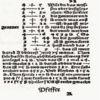### Mathematical Symbols

##### Age 5 to 14

A brief article written for pupils about mathematical symbols.### Cinema Problem

##### Age 11 to 14Challenge Level

A cinema has 100 seats. Show how it is possible to sell exactly 100 tickets and take exactly Â£100 if the prices are Â£10 for adults, 50p for pensioners and 10p for children.### Kids

##### Age 11 to 14Challenge Level

Find the numbers in this sum### 3388

##### Age 11 to 14Challenge Level

Using some or all of the operations of addition, subtraction, multiplication and division and using the digits 3, 3, 8 and 8 each once and only once make an expression equal to 24.### Making Sense of Positives and Negatives

##### Age 11 to 14

This article suggests some ways of making sense of calculations involving positive and negative numbers.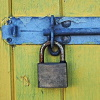### Cryptarithms

##### Age 11 to 14Challenge Level

Can you crack these cryptarithms?### Pair Sums

##### Age 11 to 14Challenge Level

Five numbers added together in pairs produce: 0, 2, 4, 4, 6, 8, 9, 11, 13, 15 What are the five numbers?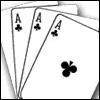### Amazing Card Trick

##### Age 11 to 14Challenge Level

How is it possible to predict the card?### Weights

##### Age 11 to 14Challenge Level

Different combinations of the weights available allow you to make different totals. Which totals can you make?##### Age 11 to 14Challenge Level

Make a set of numbers that use all the digits from 1 to 9, once and once only. Add them up. The result is divisible by 9. Add each of the digits in the new number. What is their sum? Now try some. . . .### Up, Down, Flying Around

##### Age 11 to 14Challenge Level

Play this game to learn about adding and subtracting positive and negative numbers### Diagonal Sums

##### Age 7 to 14Challenge Level

In this 100 square, look at the green square which contains the numbers 2, 3, 12 and 13. What is the sum of the numbers that are diagonally opposite each other? What do you notice?### Making a Difference

##### Age 11 to 14Challenge Level

How many different differences can you make?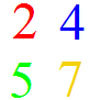### And So on and So On

##### Age 11 to 14Challenge Level

If you wrote all the possible four digit numbers made by using each of the digits 2, 4, 5, 7 once, what would they add up to?### Can You Make 100?

##### Age 11 to 14Challenge Level

How many ways can you find to put in operation signs (+ - x Ã·) to make 100?### Got it Article

##### Age 7 to 14

This article gives you a few ideas for understanding the Got It! game and how you might find a winning strategy.### Countdown Fractions

##### Age 11 to 16Challenge Level

Here is a chance to play a fractions version of the classic Countdown Game.### Crossed Ends

##### Age 11 to 14Challenge Level

Crosses can be drawn on number grids of various sizes. What do you notice when you add opposite ends?### Making Maths: Double-sided Magic Square

##### Age 7 to 14Challenge Level

Make your own double-sided magic square. But can you complete both sides once you've made the pieces?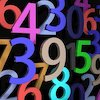### Countdown

##### Age 7 to 14Challenge Level

Here is a chance to play a version of the classic Countdown Game.### Nice or Nasty

##### Age 7 to 14Challenge Level

There are nasty versions of this dice game but we'll start with the nice ones...##### Age 11 to 14Challenge Level

If you take a three by three square on a 1-10 addition square and multiply the diagonally opposite numbers together, what is the difference between these products. Why?### 2010: A Year of Investigations

##### Age 5 to 14

This article for teachers suggests ideas for activities built around 10 and 2010.### Escape from the Castle

##### Age 7 to 14Challenge Level

Skippy and Anna are locked in a room in a large castle. The key to that room, and all the other rooms, is a number. The numbers are locked away in a problem. Can you help them to get out?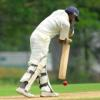### Twenty20

##### Age 7 to 16Challenge Level

Fancy a game of cricket? Here is a mathematical version you can play indoors without breaking any windows.##### Age 11 to 14Challenge Level

By selecting digits for an addition grid, what targets can you make?### Got it for Two

##### Age 7 to 14Challenge Level

Got It game for an adult and child. How can you play so that you know you will always win?### Nice or Nasty for Two

##### Age 7 to 14Challenge Level

Some Games That May Be Nice or Nasty for an adult and child. Use your knowledge of place value to beat your opponent.### Subtraction Surprise

##### Age 7 to 14Challenge Level

Try out some calculations. Are you surprised by the results?##### Age 11 to 14Challenge Level

What happens when you add a three digit number to its reverse?### Double Digit

##### Age 11 to 14Challenge Level### Consecutive Seven

##### Age 11 to 14Challenge Level

Can you arrange these numbers into 7 subsets, each of three numbers, so that when the numbers in each are added together, they make seven consecutive numbers?### Pairs

##### Age 11 to 14Challenge Level

Ann thought of 5 numbers and told Bob all the sums that could be made by adding the numbers in pairs. The list of sums is 6, 7, 8, 8, 9, 9, 10,10, 11, 12. Help Bob to find out which numbers Ann was. . . .### The Patent Solution

##### Age 11 to 14Challenge Level

A combination mechanism for a safe comprises thirty-two tumblers numbered from one to thirty-two in such a way that the numbers in each wheel total 132... Could you open the safe?### An Introduction to Magic Squares

##### Age 7 to 16### Score

##### Age 11 to 14Challenge Level

There are exactly 3 ways to add 4 odd numbers to get 10. Find all the ways of adding 8 odd numbers to get 20. To be sure of getting all the solutions you will need to be systematic. What about. . . .### Alphabet Soup

##### Age 11 to 14Challenge Level

This challenge is to make up YOUR OWN alphanumeric. Each letter represents a digit and where the same letter appears more than once it must represent the same digit each time.### As Easy as 1,2,3

##### Age 11 to 14Challenge Level

When I type a sequence of letters my calculator gives the product of all the numbers in the corresponding memories. What numbers should I store so that when I type 'ONE' it returns 1, and when I type. . . .### Football Sum

##### Age 11 to 14Challenge Level

Find the values of the nine letters in the sum: FOOT + BALL = GAME### Connect Three

##### Age 11 to 16Challenge Level

In this game the winner is the first to complete a row of three. Are some squares easier to land on than others?### Cayley

##### Age 11 to 14Challenge Level

The letters in the following addition sum represent the digits 1 ... 9. If A=3 and D=2, what number is represented by "CAYLEY"?### Two and Two

##### Age 11 to 16Challenge Level

How many solutions can you find to this sum? Each of the different letters stands for a different number.### Digit Sum

##### Age 14 to 16 ShortChallenge Level

What is the sum of all the digits in all the integers from one to one million?### Number Daisy

##### Age 11 to 14Challenge Level

Can you find six numbers to go in the Daisy from which you can make all the numbers from 1 to a number bigger than 25?### Aba

##### Age 11 to 14Challenge Level

In the following sum the letters A, B, C, D, E and F stand for six distinct digits. Find all the ways of replacing the letters with digits so that the arithmetic is correct.### Tis Unique

##### Age 11 to 14Challenge Level

This addition sum uses all ten digits 0, 1, 2...9 exactly once. Find the sum and show that the one you give is the only possibility.